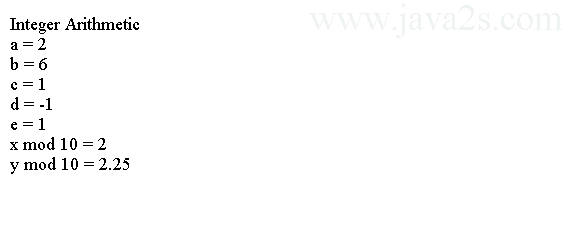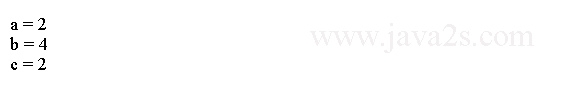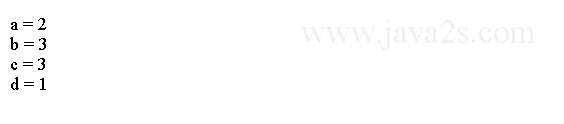# Java Tutorial - Java Arithmetic Operators

Arithmetic operators are used in mathematical expressions.

## All Arithmetic Operators

The following table lists the arithmetic operators:

OperatorResult
- Subtraction (unary minus)
* Multiplication
/ Division
% Modulus
++ Increment
-= Subtraction assignment
*= Multiplication assignment
/= Division assignment
%= Modulus assignment
-- Decrement

The operands of the arithmetic operators must be of a numeric type. You cannot use arithmetic operators on `boolean` types, but you can use them on `char` types.

The basic arithmetic operations are addition, subtraction, multiplication, and division. They behave as you would expect. The minus operator also has a unary form which negates its single operand.

The quick demo below shows how to do a simple calculation in Java with basic arithmetic operators.

```
public class Main {
//from w  ww .j  a  v  a 2 s.  c om
public static void main(String args[]) {

System.out.println("Integer Arithmetic");
int a = 1 + 1;
int b = a * 3;
int c = b / 4;
int d = c - a;
int e = -d;
System.out.println("a = " + a);
System.out.println("b = " + b);
System.out.println("c = " + c);
System.out.println("d = " + d);
System.out.println("e = " + e);

int x = 42;
System.out.println("x mod 10 = " + x % 10);
double y = 42.25;

System.out.println("y mod 10 = " + y % 10);
}
}
```

When you run this program, you will see the following output:The modulus operator, `%`, returns the remainder of a division operation. The modulus operator can be applied to floating-point types as well as integer types.

## Java Compound Assignment Operators

Statements like the following

```a = a + 4;
```

can be rewritten as

```a += 4;
```

Both statements perform the same action: they increase the value of `a` by 4.

Any statement of the form

```var = var op expression;
```

can be rewritten as

```var op= expression;
```

Here is a sample program that shows several `op=` operator assignments:

```
public class Main {
/*from  w  w  w.j  av  a2 s .co  m*/
public static void main(String args[]) {
int a = 1;
int b = 2;
int c = 3;

a += 1;
b *= 2;
c += a * b;
c %= 3;
System.out.println("a = " + a);
System.out.println("b = " + b);
System.out.println("c = " + c);

}
}
```

The output of this program is shown here:## Java Increment and Decrement Operator

`++` and `--` are Java's increment and decrement operators. The increment operator, `++`, increases its operand by one. The decrement operator, `--`, decreases its operand by one.

Different between Increment and Decrement Operator:

For example, this statement:

```x = x + 1;
```

can be rewritten like this by use of the increment operator:

```x++;
```

This statement:

```x = x - 1;
```

is equivalent to

```x--;
```

The increment and decrement operators are unique in that they can appear both in postfix form and prefix form. In the postfix form they follow the operand, for example, `i++`. In the prefix form, they precede the operand, for example, `--i`.

The difference between these two forms appears when the increment and/or decrement operators are part of a larger expression. In the prefix form, the operand is incremented or decremented before the value is used in the expression. In postfix form, the value is used in the expression, and then the operand is modified.

The following table summarizes the difference between Pre-and Post- Increment and Decrement Operations:

Initial Value of xExpressionFinal Value of yFinal Value of x
5 y = x++ 5 6
5 y = ++x 6 6
5 y = x-- 5 4
5 y = --x 4 4

For example:

```x = 42;
y = ++x;
```

`y` is set to 43, because the increment occurs before x is assigned to y. Thus, the line

```y = ++x;
```

is the equivalent of these two statements:

```x = x + 1;
y = x;
```

However, when written like this,

```x = 42;
y = x++;
```

the value of x is obtained before the increment operator is executed, so the value of y is 42.

In both cases x is set to 43. The line

```y = x++;
```

is the equivalent of these two statements:

```y = x;
x = x + 1;
```

The following program demonstrates the increment operator.

```
public class Main {
/* ww  w  .ja v  a2s .c o  m*/
public static void main(String args[]) {
int a = 1;
int b = 2;
int c = ++b;
int d = a++;

System.out.println("a = " + a);
System.out.println("b = " + b);
System.out.println("c = " + c);
System.out.println("d = " + d);

}
}
```

The output of this program follows: